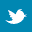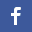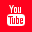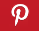Completely Solved C, C++ Programs Assignment.Quick Search Database Videos Tutorials Ebooks Forums FAQ Aboutus Household Industrial Manufacturing Service Shopping Transportation### Basic C Program

 Filed Under:

 Basic C Program:

# write a program in C to find the area of triangle .

Write a program in C to find the area of sphere
Write a program in C to find the circumference of circle

# Write a program in C to find the area of circle using input value

Write a program in C to find the area of triangle using input value
Write a program in C to find the area of sphere using input value

# Write a program in C to find the circumference of circle using input value

Write a program in C to read and print an given character
Write a program in C to print the ascii value of given character

# Write a program in C to read and print the given integer

Write a program in C to read and print the given float

# Write a program in C to Arithmetic operations of two integer numbers .Print these results in separate lines

Write a program in C to Arithmetic operations of two long numbers . Print these results in separate      lines

# Write a program in C to read float value and print integer equivalent

Write a program in C to read int value and print float equivalent